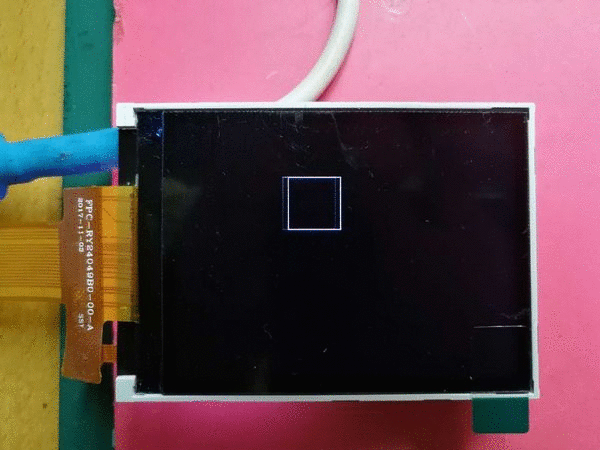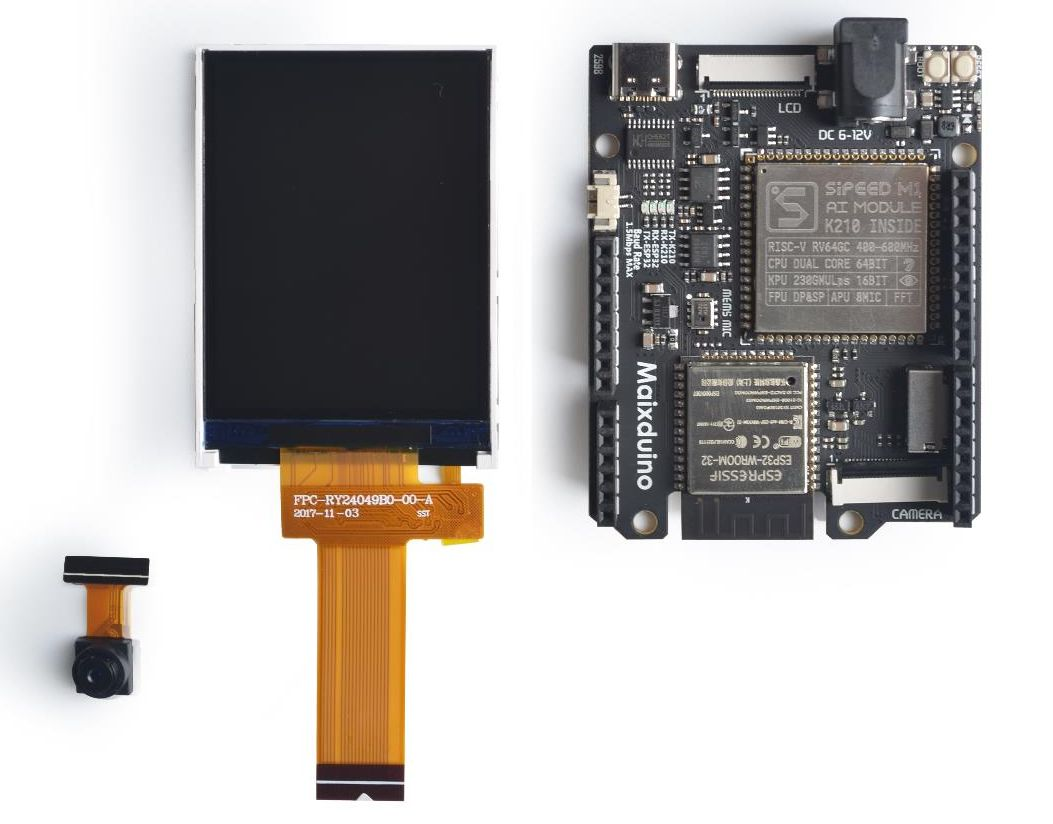ST7789驱动器芯片2.4寸LCD屏（24P 320X240）

1. 模块名称：液晶显示模块
2. 型号：KD024C-4
3. 同类型型号:
4. 兼容型号：
5. 显示模式：TFT
6. 显示色彩：65/262K
7. 分辨率: 240*320
8. 点距：0.153 (H) x 0.153 (V)
9. 视角：12:00
10. 控制IC：ST7789V
11. 显示类型：全透型，常白
12. 外形尺寸：42.72*60.26*2.6mm
13. 可视面积：38.32*50.56 mm
14. 点阵区面积：36.72*48.96mm
15. 亮度：300cd/m2
16. 对比度：500
17. 接口类型: 8/9/16/18位8080并口
16/18位RGB接口
3/4线SPI接口
18. 引脚数：24
19. 引脚距离：0.5mm
20. 连接类型: FPC插接型
21. 工作电压：3.3V
22. 背光灯颜色及类型: 白色LED背光
23. 背光电路：4 LED 并联, 共阳
If=80mA, Vf =3.2V
24. 使用寿命：100000h
25. 工作温度：-20----70°C
26. 储存温度：-30----80°C
27. 质量体系认证：ISO9001:2008
28. 产品认证：RoHS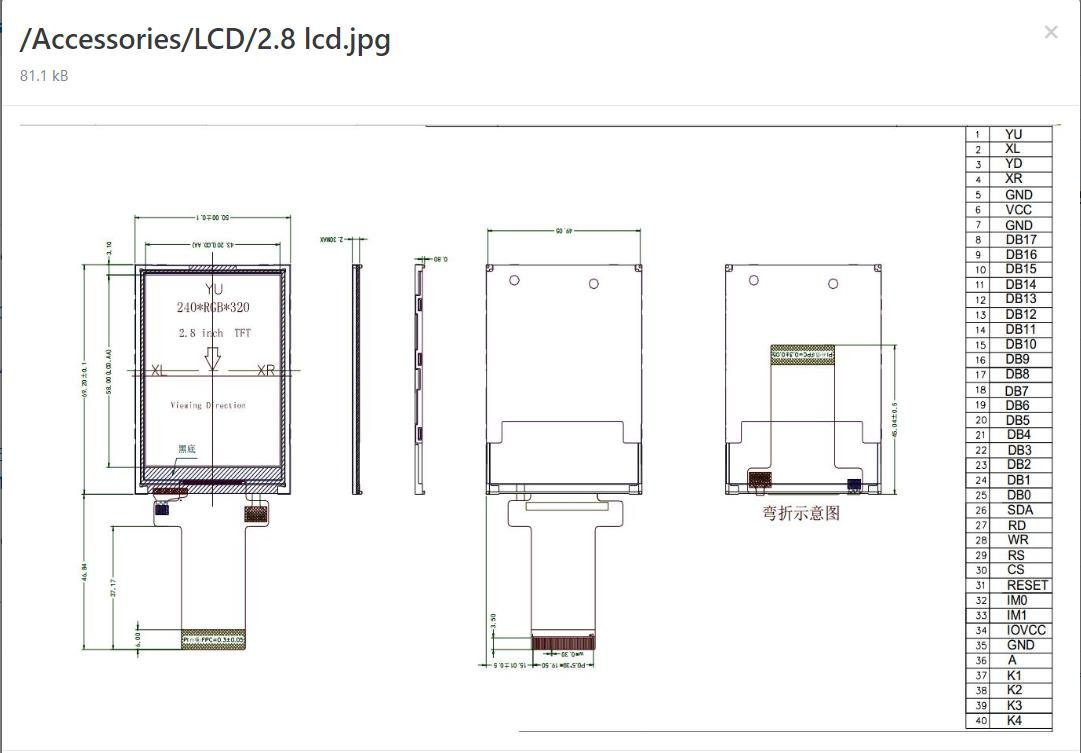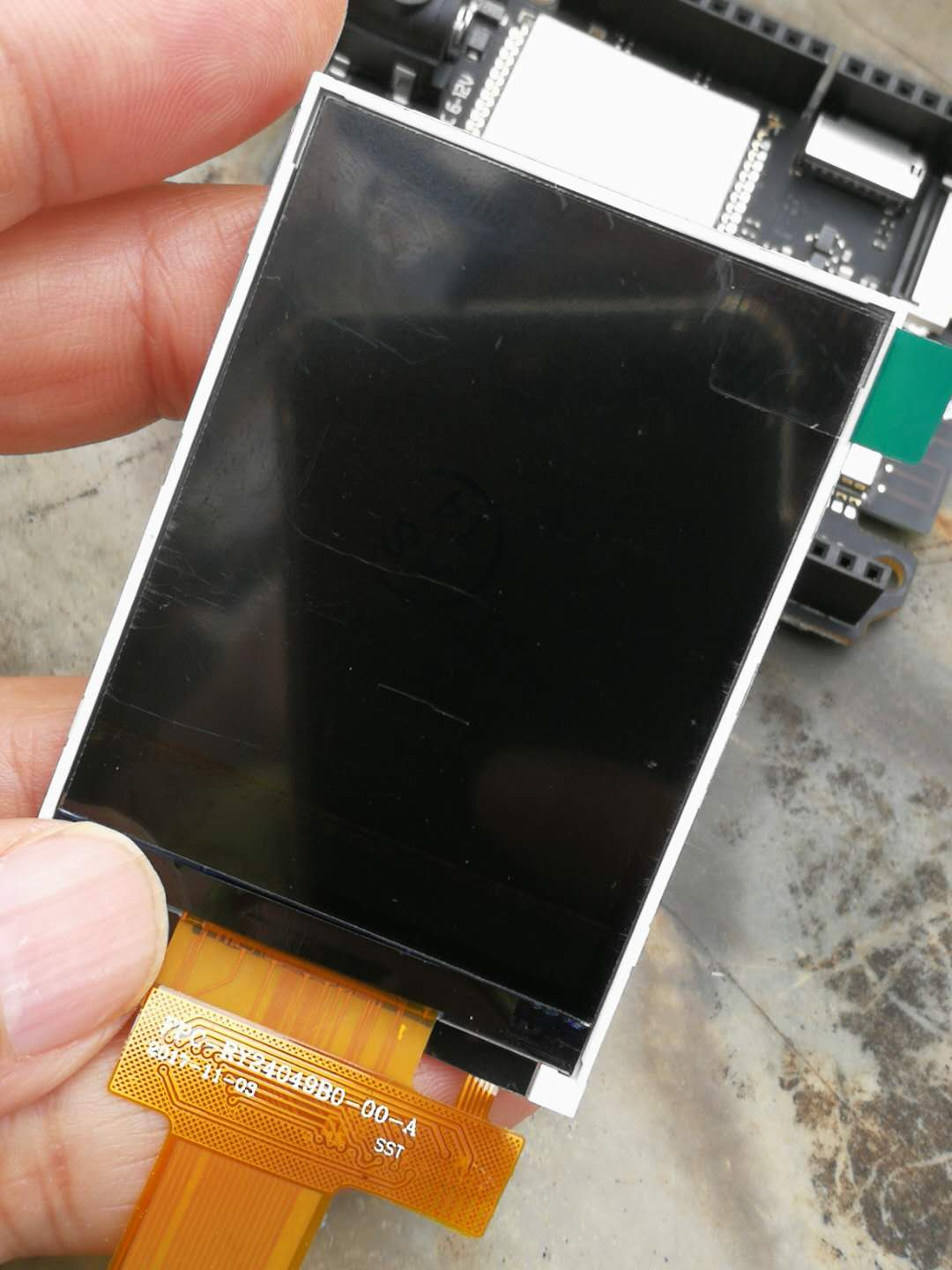#MicroPython动手做（05）——零基础学MaixPy之LCD液晶屏
#测试程序之一：显示英文

#MicroPython动手做（05）——零基础学MaixPy之LCD液晶屏

#测试程序之一：显示英文

import lcd

lcd.init()

lcd.draw_string(100, 100, "hello MicroPython!", lcd.RED, lcd.BLACK)

lcd 屏幕显示驱动

lcd.display(image, roi=Auto)

roi 是一个感兴趣区域的矩形元组(x, y, w, h)。若未指定，即为图像矩形

roi 是键值参数，必须在函数调用中通过写入 roi= 来显式地调用。

#MicroPython动手做（05）——零基础学MaixPy之LCD液晶屏

#测试程序之二：LCD屏显示"hello maixpy"

import lcd, time

import image

bg = (236,36,36)

lcd.init(freq=15000000)

lcd.direction(lcd.YX_RLDU)

lcd.clear(lcd.RED)

time.sleep(1)

lcd.draw_string(120, 120, "hello maixpy", lcd.WHITE, lcd.RED)

time.sleep(2)

img = image.Image()

img.draw_string(60, 100, "hello maixpy", scale=2)

img.draw_rectangle((120,120,30,30))

lcd.display(img)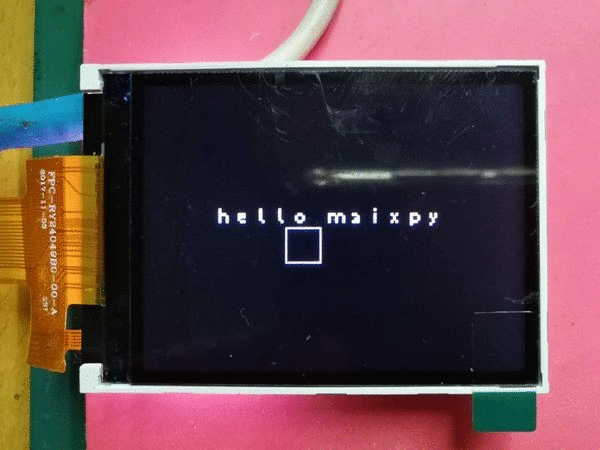lcd 屏幕显示驱动函数1. lcd.init(type=1, freq=15000000, color=lcd.BLACK)初始化 LCD 屏幕显示参数type： LCD 的类型（保留给未来使用）:0: None1: lcd shield（默认值）type 是键值参数，必须在函数调用中通过写入 type= 来显式地调用freq： LCD （实际上指 SPI 的通讯速率） 的频率color： LCD 初始化的颜色， 可以是 16 位的 RGB565 颜色值，比如 0xFFFF； 或者 RGB888 元组， 比如 (236, 36, 36)， 默认 lcd.BLACK2. lcd.clear()将液晶屏清空为黑色或者指定的颜色。参数color： LCD 初始化的颜色， 可以是 16 位的 RGB565 颜色值，比如 0xFFFF； 或者 RGB888 元组， 比如 (236, 36, 36)3. lcd.freq(freq)设置或者获取 LCD （SPI） 的频率Paremetersfreq: LCD (SPI) 的频率ReturnLCD 的频率4. lcd.direction(dir)在 v0.3.1 之后已经被舍弃， 请使用lcd.rotation 和 lcd.invert代替， 如非必要请勿使用， 接口仍会被保留用于调试使用设置屏幕方向， 以及是否镜像等参数dir： 正常情况下推荐 lcd.YX_LRUD 和 lcd.YX_RLDU， 另外还有其它值，交换 XY 或者 LR 或者 DU即可
#MicroPython动手做（05）——零基础学MaixPy之LCD液晶屏

#实验程序之三：LCD液晶显示器彩条测试

import lcd,image, time

lcd.init(type=1, freq=15000000)

# lcd.init(type=2, freq=20000000)

# lcd.init(type=1, width=320, height=240, invert=True, freq=20000000)

img = image.Image(size=(240,240))

img.draw_rectangle(0,0,30, 240, fill=True, color=(0xff, 0xff, 0xff))

img.draw_rectangle(30,0,30, 240, fill=True, color=(250, 232, 25))

img.draw_rectangle(60,0,30, 240, fill=True, color=(106, 198, 218))

img.draw_rectangle(90,0,30, 240, fill=True, color=(98, 177, 31))

img.draw_rectangle(120,0,30, 240, fill=True, color=(180, 82, 155))

img.draw_rectangle(150,0,30, 240, fill=True, color=(231, 47, 29))

img.draw_rectangle(180,0,30, 240, fill=True, color=(32, 77, 158))

img.draw_rectangle(210,0,30, 240, fill=True, color=(27, 28, 32))

lcd.display(img)

count = 500

while count > 0:

t = time.ticks_ms()

lcd.display(img)

print(time.ticks_ms() - t)

count -= 1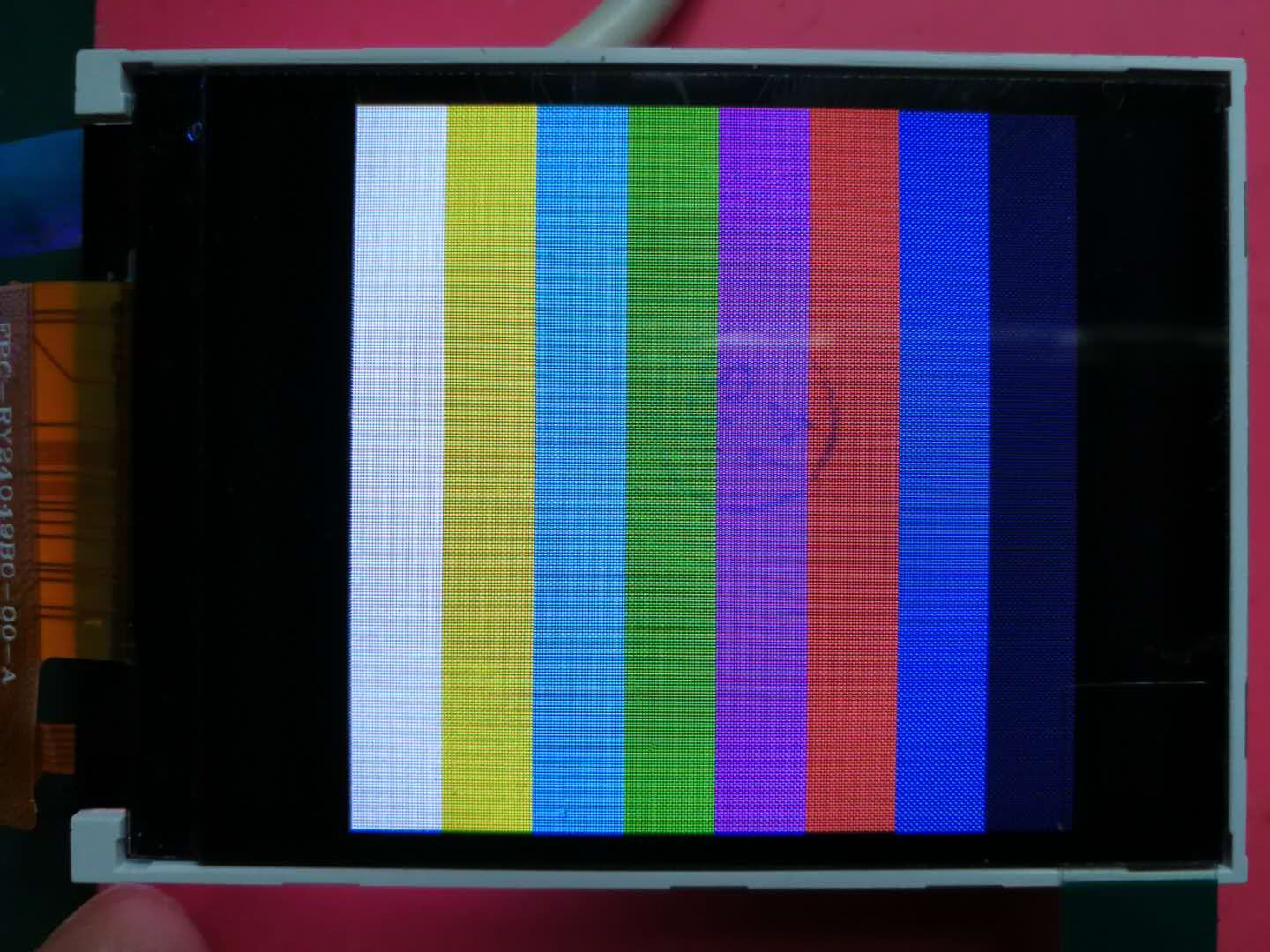#MicroPython动手做（05）——零基础学MaixPy之LCD液晶屏
#实验程序之四：测试Microphone阵列算法

from Maix import MIC_ARRAY as mic
import lcd

lcd.init()
mic.init()

while True:
imga = mic.get_map()
b = mic.get_dir(imga)
a = mic.set_led(b,(0,0,255))
imgb = imga.resize(160,160)
imgc = imgb.to_rainbow(1)
a = lcd.display(imgc)
mic.deinit()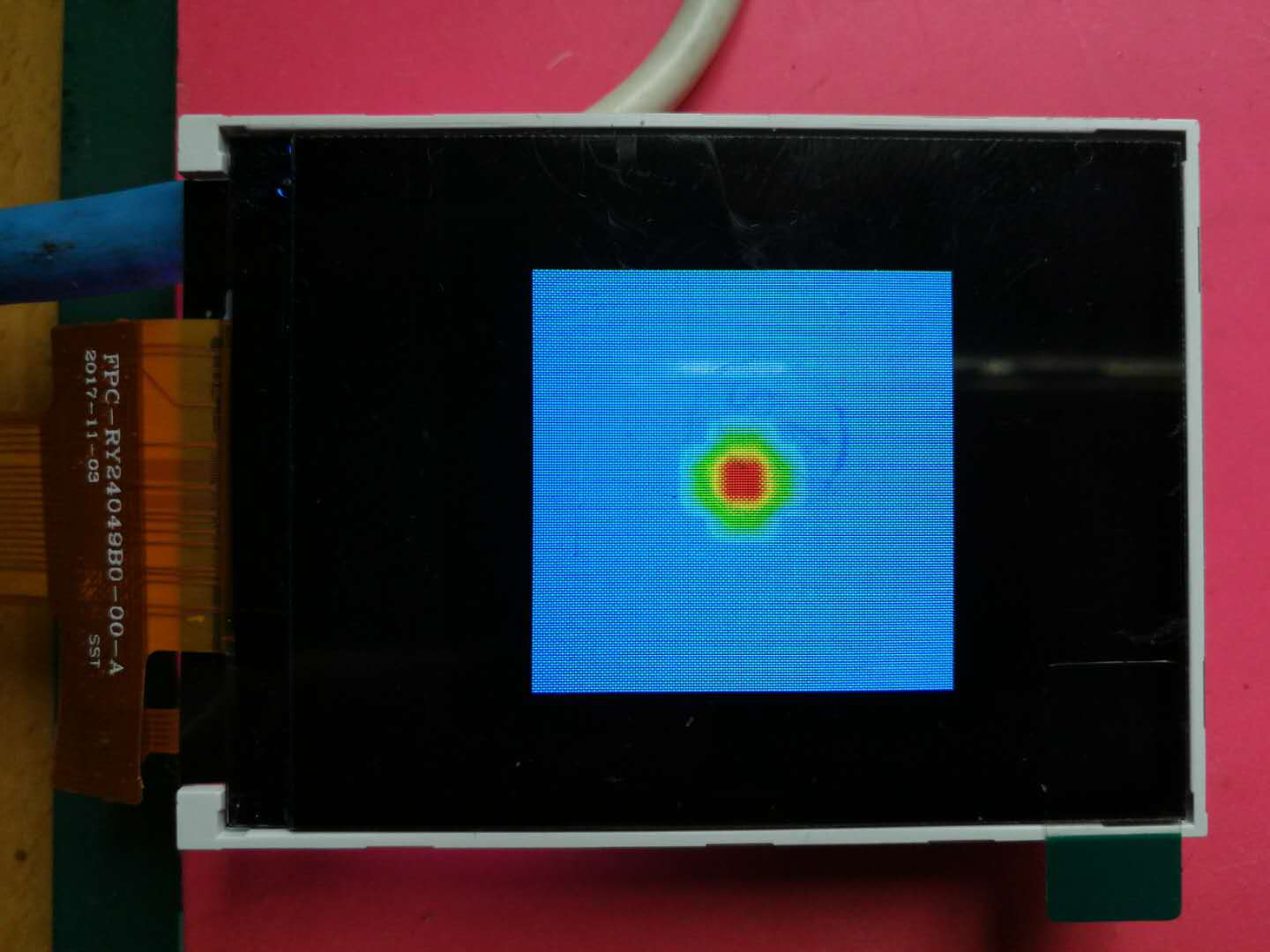#MicroPython动手做（05）——零基础学MaixPy之LCD液晶屏

#实验程序之五：图形与hello maixpy

import lcd, time

import image

bg = (236,36,36)

lcd.init(freq=15000000)

lcd.direction(lcd.YX_RLDU)

img = image.Image()

img.draw_string(60, 100, "hello maixpy", scale=2)

img.draw_rectangle((120,120,30,30))

img.draw_circle((150,140, 80))

img.draw_cross((250,40))

img.draw_arrow((250,200,20,200), color=(236,36,36))

lcd.display(img)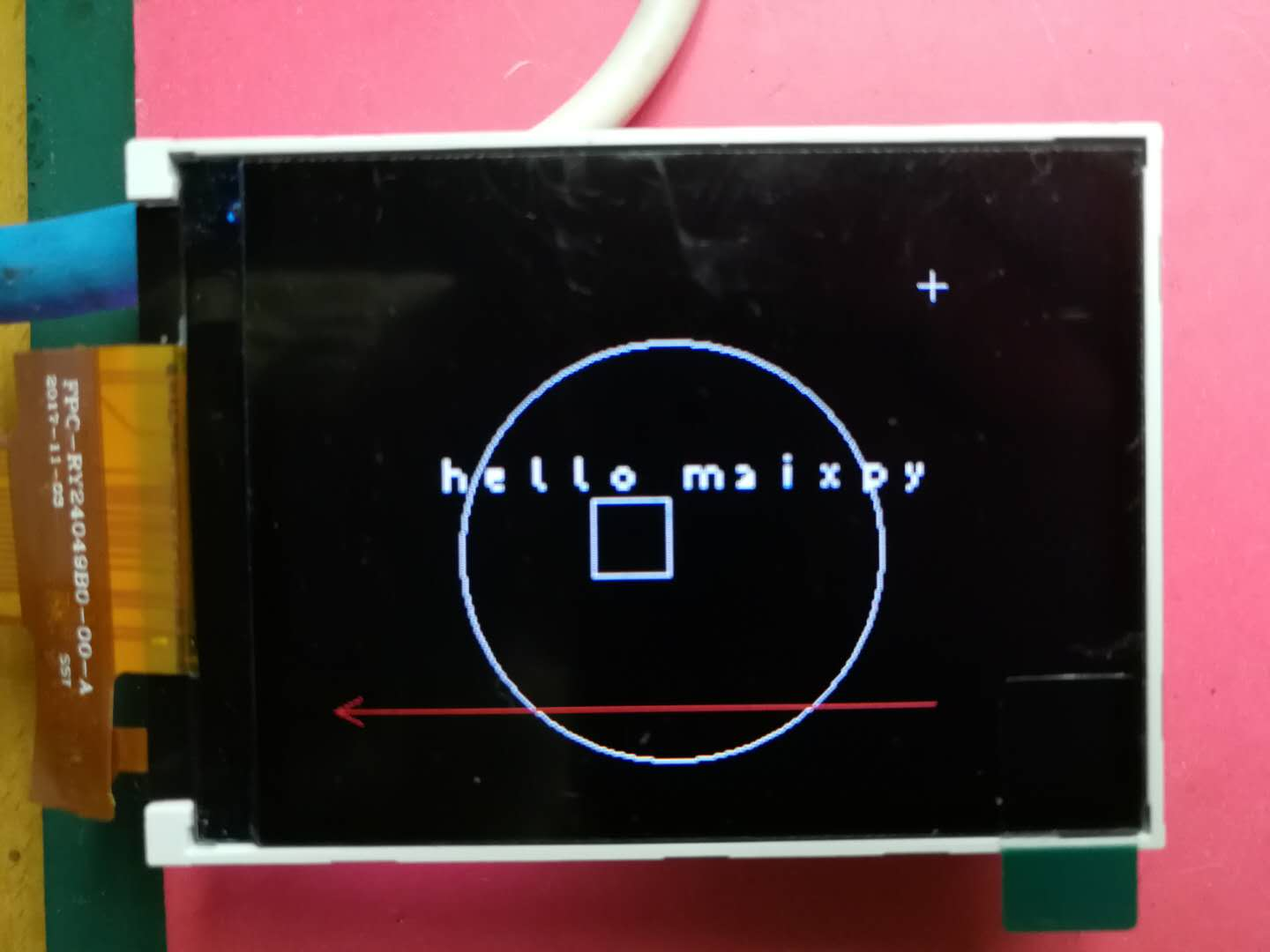#MicroPython动手做（05）——零基础学MaixPy之LCD液晶屏

#实验程序之六：碰碰球演示

import image, lcd

lcd.init(freq=20000000)

i = 0

dir = 1

while(True):

img = image.Image(copy_to_fb=1)

img.clear()

img.draw_rectangle(i,50,50,50)

lcd.display(img)

if dir:

i += 5

if i==270:

dir = 0

else:

i -= 5

if i==0:

dir = 1